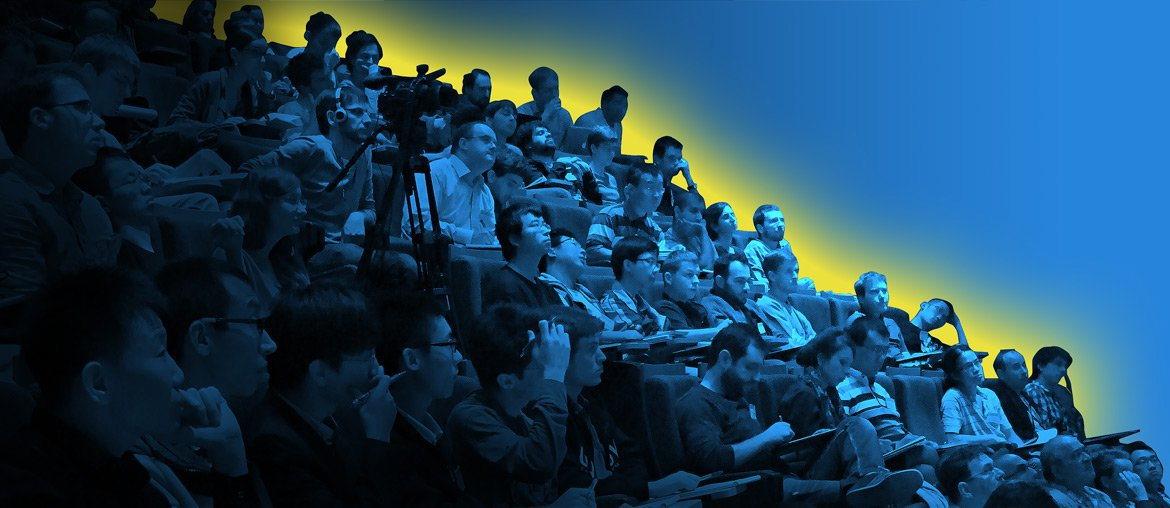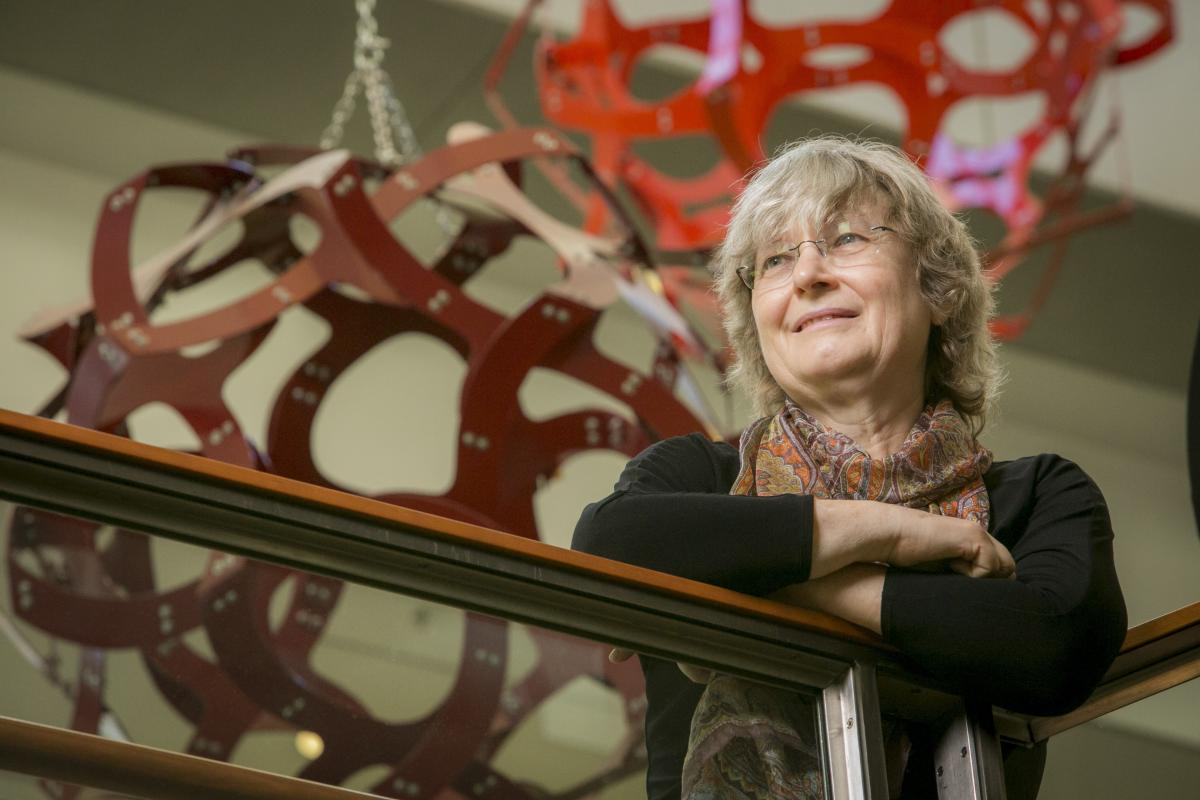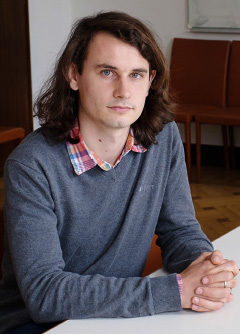• Accueil

• La FMJH Organise

• Evénements

# 2012-2018

## 2018 Ingrid DAUBECHIES

### "Time-Frequency Localization and Applications"In this 250th anniversary year of the birth of Joseph Fourier, it behoves us to talk of frequency and spectral analysis!

The lectures shall visit a number of different techniques that have been developed and applied in the last 30 years, to carry out what engineers and applied mathematicians commonly call time-frequency analysis; in different settings, this approach also goes by the name micro-local analysis. The goal is to decompose signals, functions and operators in ways that preserve, isolate or emphasize local features in both time (or space) and frequency (or momentum). Decompositions of this type can be viewed as analogous to standard music notation, which tells the musician which notes (= frequency information) to play when (= localization in time).

Tools used for time-frequency localization include, for instance, the so-called short-time Fourier transform as well as wavelets and curvelets; both tools have applications that range widely, and that include, to name a few, semiclassical approximations and estimates in quantum mechanics, image compression, new tools for art conservators, and filters used for gravitational wave detection. We will also discuss the important role of sparsity, a central concept not only in signal analysis (compressed sensing) but also in inverse problems and large-scale computation.

## 2017 Peter SCHOLZE

### "On the local Langlands conjectures for reductive groups over p-adic fields"Consider a reductive group G over a p-adic field F. The local Langlands conjecture relates the irreducible smooth representations of G(F) with the set of (local) L-parameters, which are maps from the Weil group of F to the L-group of G; refinements of the conjecture relate the fibres of this map with the automorphism group of the L-parameter. Based on ideas from V. Lafforgue's work in the global function field case, I outlined a strategy for attaching (semisimple) L-parameters to irreducible smooth representations of G(F) in my 2014 Berkeley course. At the same time and place, L. Fargues formulated a conjecture relating the local Langlands conjecture with a geometric Langlands conjecture on the Fargues-Fontaine curve. The goal of this course will be to discuss some of the developments since then. On the foundational side, this concerns basics on the etale cohomology of diamonds including smooth and proper base change and Poincare duality, leading up to a good notion of "constructible" sheaves on the stack of G-bundles on the Fargues-Fontaine curve. On the applied side, this concerns the construction of (semisimple) L-parameters, the conjecture of Harris (as modified by Viehmann) on the cohomology of non-basic Rapoport-Zink spaces, and the conjecture of Kottwitz on the cohomology of basic Rapoport-Zink spaces.

## 2016 Carlos KENIG

### "The energy critical wave equation"The theory of nonlinear dispersive equations has seen a tremendous development in the last 35 years. The initial works studied the behavior of special solutions such as traveling waves and solitons. Then, there was a systematic study of the well-posedness theory (in the sense of Hadamard) using extensively tools from harmonic analysis. This yielded many optimal results on the short-time well-posedness and small data global well-posedness of many classical problems. The last 25 years have seen a lot of interest in the study, for nonlinear dispersive equations, of the long-time behavior of solutions, for large data. Issues like blow-up, global existence, scattering and long-time asymptotic behavior have come to the forefront, especially in critical problems. In these lectures we will concentrate on the energy critical nonlinear wave equation, in the focusing case. The dynamics in the defocusing case were studied extensively in the period 1990-2000, culminating in the result that all large data in the energy space yield global solutions which scatter. The focusing case is very different since one can have finite time blow-up, even for solutions which remain bounded in the energy norm, and solutions which exist and remain bounded in the energy norm for all time, but do not scatter, for instance traveling wave solutions, and other fascinating nonlinear phenomena. In these lectures I will explain the progress in the last 10 years, in the program of obtaining a complete understanding of the dynamics of solutions which remain bounded in the energy space. This has recently led to a proof of soliton resolution, in the non-radial case, along a well-chosen sequence of times. This will be one of the highlights of the lectures. It is hoped that the results obtained for this equation will be a model for what to strive for in the study of other critical nonlinear dispersive equations.

## 2015 Martin HAIRER

### "Regularity Structures"One of the main challenges of modern mathematical physics is to understand the behaviour of systems at or near criticality. In a number of cases, one can argue heuristically that this behaviour should be described by a nonlinear stochastic partial differential equation. Some examples of systems of interest are models of phase coexistence near the critical temperature, one-dimensional interface growth models, and models of absorption of a diffusing particle by random impurities. Unfortunately, the equations arising in all of these contexts are mathematically ill-posed to the extent that they defeat classical stochastic PDE techniques.
Recently, the theory of regularity structures has allowed us to give a rigorous mathematical interpretation to such equations and to build the mathematical objects conjectured to describe the abovementioned systems near criticality.
It also comes with a robust solution theory allowing to prove various approximation results by solutions to classical PDEs, possibly with diverging coefficients. The aim of these lectures is to give an overview of the main results and concepts of the theory. Whenever practical, we will give at least sketches of proofs that are as self-contained as possible.

## 2014 Ben GREEN

### « Nilsequences »Classical Fourier analysis has found many uses in additive number theory. However, while it is well-adapted to some problems, it is unable to handle others. For example, if one has a set A, and one wishes to know how many 3-term arithmetic progressions are contained in A, then Fourier analysis is useful, but if one wishes to count 4-term progressions then it is not. For this, and other, problems the more general notion of a nilsequence is required. NIlsequences are a kind of «higher order character» forming the basis of what is becoming known as «higher-order Fourier analysis». The talks will be about this theory.

## 2012 Pr. Victor KAK et Felix OTTO